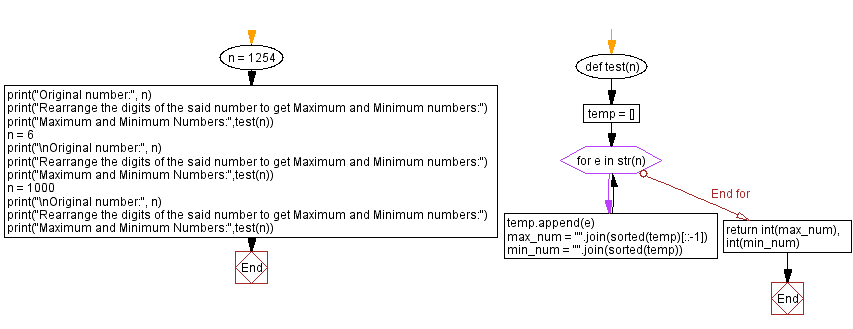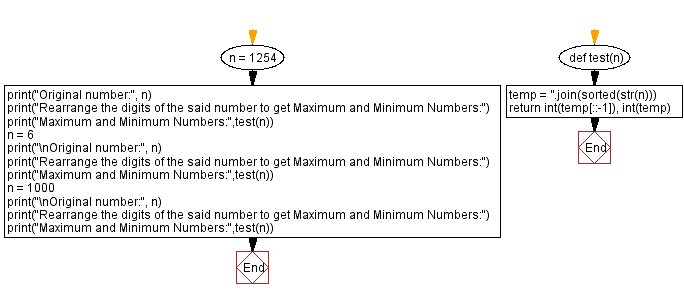﻿ Python - Rearrange the digits of a number# Python Exercises: Rearrange the digits of a number

## Python Math: Exercise-93 with Solution

Write a Python program to that takes an integer and rearrange the digits to create two maximum and minimum numbers.

Sample Solution-1:

Python Code:

``````def test(n):
temp = []
for e in str(n):
temp.append(e)
max_num = "".join(sorted(temp)[::-1])
min_num = "".join(sorted(temp))
return int(max_num), int(min_num)

n = 1254
print("Original number:", n)
print("Rearrange the digits of the said number to get Maximum and Minimum numbers:")
print("Maximum and Minimum Numbers:",test(n))
n = 6
print("\nOriginal number:", n)
print("Rearrange the digits of the said number to get Maximum and Minimum numbers:")
print("Maximum and Minimum Numbers:",test(n))
n = 1000
print("\nOriginal number:", n)
print("Rearrange the digits of the said number to get Maximum and Minimum numbers:")
print("Maximum and Minimum Numbers:",test(n))
```
```

Sample Output:

```Original number: 1254
Rearrange the digits of the said number to get Maximum and Minimum numbers:
Maximum and Minimum Numbers: (5421, 1245)

Original number: 6
Rearrange the digits of the said number to get Maximum and Minimum numbers:
Maximum and Minimum Numbers: (6, 6)

Original number: 1000
Rearrange the digits of the said number to get Maximum and Minimum numbers:
Maximum and Minimum Numbers: (1000, 1)
```

Flowchart:## Visualize Python code execution:

The following tool visualize what the computer is doing step-by-step as it executes the said program:

Sample Solution-2:

Python Code:

``````def test(n):
temp = ''.join(sorted(str(n)))
return int(temp[::-1]), int(temp)

n = 1254
print("Original number:", n)
print("Rearrange the digits of the said number to get Maximum and Minimum Numbers:")
print("Maximum and Minimum Numbers:",test(n))
n = 6
print("\nOriginal number:", n)
print("Rearrange the digits of the said number to get Maximum and Minimum Numbers:")
print("Maximum and Minimum Numbers:",test(n))
n = 1000
print("\nOriginal number:", n)
print("Rearrange the digits of the said number to get Maximum and Minimum Numbers:")
print("Maximum and Minimum Numbers:",test(n))
```
```

Sample Output:

```Original number: 1254
Rearrange the digits of the said number to get Maximum and Minimum Numbers:
Maximum and Minimum Numbers: (5421, 1245)

Original number: 6
Rearrange the digits of the said number to get Maximum and Minimum Numbers:
Maximum and Minimum Numbers: (6, 6)

Original number: 1000
Rearrange the digits of the said number to get Maximum and Minimum Numbers:
Maximum and Minimum Numbers: (1000, 1)
```

Flowchart:## Visualize Python code execution:

The following tool visualize what the computer is doing step-by-step as it executes the said program:

Python Code Editor:

Have another way to solve this solution? Contribute your code (and comments) through Disqus.

Previous Python Exercise: Absolute difference between two consecutive digits.
Next Python Exercise: Sum of all prime numbers in a list of integers.

What is the difficulty level of this exercise?

Test your Programming skills with w3resource's quiz.

﻿

## Python: Tips of the Day

Getting sorted iterable (can sort by "compare" function):

```>>> a = [1, 2, -3]
>>> sorted(a)
[-3, 1, 2]

>>> sorted(a,key=abs)
[1, 2, -3]
```